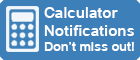Calculator Library
Free Online CalculatorsHome » 87 Calculators » Other » Percent Calculator

Huge Domain Name Sale | Free Domains
Domain names on sale at crazy prices. Register a new domain for FREE when you buy another service.

## PERCENT CALCULATOR

INSTRUCTIONS

This calculator is designed to perform percentage calculations and help you work out various values related to percentages.

1. Enter the relevant data in the first two fields of each calculation.
2. When you are finished entering the data, click the Calculate button next to your desired result to calculate that value.

Enter Data Here

1. Calculate percentage of a number:
% of =

2. Calculate what percentage one number is of another:
as a % of = %

3. Calculate percentage variation from one number to another:
% variation from to = %

#### NOTES

1. Percentages are ratios that represent fractions of 100, so the word "percent" really means "per 100".
2. The basic formula to calculate percentages is % = (Value ÷ Total Value) × 100.
3. A value written as a percentage can also be written as a decimal or a fraction. For example, we know that a quarter is 25% but this can also be expressed as 0.25 or 25100 which reduces to 14.